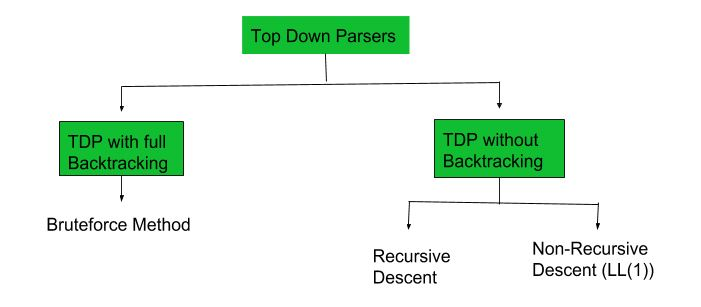GeeksforGeeks App
Open AppBrowser
Continue

# Construction of LL(1) Parsing Table

Prerequisite – Classification of top-down parsers, FIRST Set, FOLLOW Set

A top-down parser builds the parse tree from the top down, starting with the start non-terminal. There are two types of Top-Down Parsers:

1. Top-Down Parser with Backtracking
2. Top-Down Parsers without Backtracking

Top-Down Parsers without backtracking can further be divided into two parts:In this article, we are going to discuss Non-Recursive Descent which is also known as LL(1) Parser.

LL(1) Parsing: Here the 1st L represents that the scanning of the Input will be done from the Left to Right manner and the second L shows that in this parsing technique, we are going to use the Left most Derivation Tree. And finally, the 1 represents the number of look-ahead, which means how many symbols are you going to see when you want to make a decision.

Essential conditions to check first are as follows:

1. The grammar is free from left recursion.
2. The grammar should not be ambiguous.
3. The grammar has to be left factored in so that the grammar is deterministic grammar.

These conditions are necessary but not sufficient for proving a LL(1) parser.

## Algorithm to construct LL(1) Parsing Table:

Step 1:  First check all the essential conditions mentioned above and go to step 2.

Step 2: Calculate First() and Follow() for all non-terminals.

1.  First(): If there is a variable, and from that variable, if we try to drive all the strings then the beginning Terminal Symbol is called the First.
2. Follow(): What is the Terminal Symbol which follows a variable in the process of derivation.

Step 3: For each production A –> α. (A tends to alpha)

1. Find First(α) and for each terminal in First(α), make entry A –> α in the table.
2. If First(α) contains ε (epsilon) as terminal, then find the Follow(A) and for each terminal in Follow(A), make entry A –>  ε in the table.
3. If the First(α) contains ε and Follow(A) contains \$ as terminal, then make entry A –>  ε in the table for the \$.
To construct the parsing table, we have two functions:

In the table, rows will contain the Non-Terminals and the column will contain the Terminal Symbols. All the Null Productions of the Grammars will go under the Follow elements and the remaining productions will lie under the elements of the First set.

Now, let’s understand with an example.

Example 1: Consider the Grammar:

```E --> TE'
E' --> +TE' | ε
T --> FT'
T' --> *FT' | ε
F --> id | (E)

*ε denotes epsilon```

Step 1: The grammar satisfies all properties in step 1.

Step 2: Calculate first() and follow().

Find their First and Follow sets:

Step 3: Make a parser table.

Now, the LL(1) Parsing Table is:

As you can see that all the null productions are put under the Follow set of that symbol and all the remaining productions lie under the First of that symbol.

Note: Every grammar is not feasible for LL(1) Parsing table. It may be possible that one cell may contain more than one production.

Let’s see an example.

Example 2: Consider the Grammar

```S --> A | a
A --> a ```

Step 1: The grammar does not satisfy all properties in step 1, as the grammar is ambiguous. Still, let’s try to make the parser table and see what happens

Step 2: Calculating first() and follow()

Find their First and Follow sets:

Step 3: Make a parser table.

Parsing Table:

Here, we can see that there are two productions in the same cell. Hence, this grammar is not feasible for LL(1) Parser.

Trick –  Above grammar is ambiguous grammar. So the grammar does not satisfy the essential conditions. So we can say that this grammar is not feasible for LL(1) Parser even without making the parse table.

Example 3: Consider the Grammar

```S -> (L) | a
L -> SL'
L' -> )SL' | ε```

Step1: The grammar satisfies all properties in step 1

Step 2: Calculating first() and follow()

Step 3: Making a parser table

Parsing Table:

Here, we can see that there are two productions in the same cell. Hence, this grammar is not feasible for LL(1) Parser. Although the grammar satisfies all the essential conditions in step 1, it is still not feasible for LL(1) Parser.  We saw in example 2 that we must have these essential conditions and in example 3 we saw that those conditions are insufficient to be a LL(1) parser.

My Personal Notes arrow_drop_up